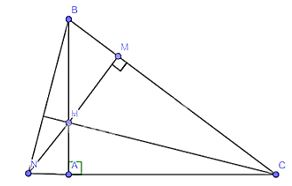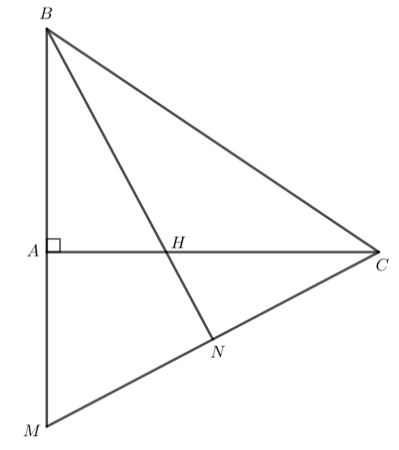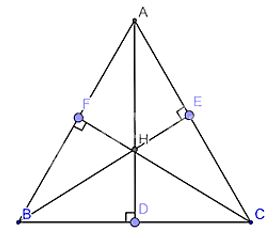## Solving Exercises Lesson 8: Properties of the three altitudes of a triangle (C8 Math 7 Horizons) – Math Book

Solving Exercises Lesson 8: Properties of the three altitudes of a triangle (C8 Math 7 Horizons)
==========

### Solution 1 page 78 Math textbook 7 Creative horizon volume 2 – CTST

Let ABC be a right triangle at A. Take the point H on side AB. Draw HM perpendicular to BC at M. Ray MH intersects ray CA at N. Prove that CH is perpendicular to NB.

Detailed instructions for solving Lesson 1

Solution method

We show that H is the orthocenter of triangle NBC

Detailed explanationSince triangle ABC is right-angled at A by assumption, BA is perpendicular to AC

Since HM intersects AC at N, HM is perpendicular to BC (assumption)

\( \Rightarrow \) NM is perpendicular to BC at USA

Consider triangle NBC with altitudes NM and BA

Since MN intersects AB at H, H is the orthocenter of triangle NBC

\( \Rightarrow \) CH altitude of triangle NBC (3 altitudes of triangle passing through 1 point)

\( \Rightarrow \) CH perpendicular to NB

–>

— *****

### Solution 2 page 78 Math textbook 7 Creative horizon volume 2 – CTST

Let ABC be a right triangle at A. On ray BA take point M such that BM = BC. The bisector of angle B intersects AC at H. Prove that MH is perpendicular to BC.

Detailed instructions for solving Lesson 2

Solution method

– We show that H is the orthocenter of triangle AMC

– From there, we prove that MH is perpendicular to BC

Detailed explanationTriangle BMC has BM = BC, so triangle BMC is isosceles at B.

The triangle BMC is isosceles at B, BN is the bisector, so BN is also the altitude of triangle BMC.

Therefore BN

$\perp$

MC.

Triangle BMC has CA

$\perp$

BM, BN

$\perp$

MC should CA, BN ​​are the two altitudes of the triangle BMC.

Since CA and BN intersect at H, H is the orthocenter of triangle BMC.

Therefore MH

$\perp$

BC.

–>

— *****

### Solve problem 3 page 78 Math textbook 7 Creative horizon volume 2 – CTST

Let ABC be an isosceles triangle at A. Take point E on side AC. On the opposite ray of ray AB take point D such that AD = AE. Prove that:

a) DE is perpendicular to BC

b) BE is perpendicular to DC

Detailed instructions for solving Lesson 3

Solution method

– We prove perpendicularity through isosceles right triangles

– We show that E is the orthocenter of triangle BCD

– Then we prove that DE is perpendicular to BC and BE is perpendicular to DC

Detailed explanationa) Since triangle ABC is right-angled at A

\( \Rightarrow \) \(\widehat B = \widehat C = {45^o}\)(2 bottom angles are equal)

Consider triangle AED with:

AC is perpendicular to AB

\( \Rightarrow \) isosceles triangle AED at A

\( \Rightarrow \widehat {ADE} = \widehat {AED} = {45^o}\)

Where \(\widehat {AED};\widehat {CEF}\) are two opposite angles \( \Rightarrow \widehat {AED} = \widehat {CEF} = {45^o}\)

Considering the triangle CEF, applying the summation theorem of 3 angles in the triangle, we have:

\( \Rightarrow \widehat F + \widehat C + \widehat E = {180^o}\)

\( \Rightarrow \widehat F = {180^o} – {45^o} – {45^o} = {90^o} \Rightarrow EF \bot BC \Rightarrow DE \bot BC\)

b) Since DE is perpendicular to BC \( \Rightarrow \) DE is altitude of triangle BCD

Since AC intersects DE at E, E is the orthocenter of triangle BCD (Since AC is also altitude of triangle BCD).

\( \Rightarrow \)BE is the altitude of triangle BCD (the theorem of 3 altitudes in a triangle passing through the orthocenter)

\( \Rightarrow \)BE perpendicular to DC

–>

— *****

### Solution 4 page 78 Math textbook 7 Creative horizon volume 2 – CTST

Let ABC be an acute triangle with three altitudes AB, BE, CF. Know AD = BE = CF. Prove that triangle ABC is equilateral.

Detailed instructions for solving Lesson 4

Solution method

– We prove triangle BFC = triangle BEC

– From that infer angle B = angle C

– Similar proof, deduce angle A = angle B = angle C

Detailed explanationConsider triangle BFC and triangle BEC:

General BC

FC = BE

\(\widehat {BFC} = \widehat {BEC} = {90^o}\)

(hypogonal side – right angle side)

\( \Rightarrow \widehat C = \widehat B\) ( 2 corresponding angles ) (1)

Considering triangle CFA and triangle ADC, we have:

Common AC

\(\widehat {ADC} = \widehat {AFC} = {90^o}\)

(hype side – right angle side)

\( \Rightarrow \widehat C = \widehat A\)(2 corresponding angles ) (2)

From (1) and (2) \( \Rightarrow \widehat C = \widehat A = \widehat B\) \( \Rightarrow \)Triangle ABC is an equilateral triangle because it has 3 equal angles

–>

— *****